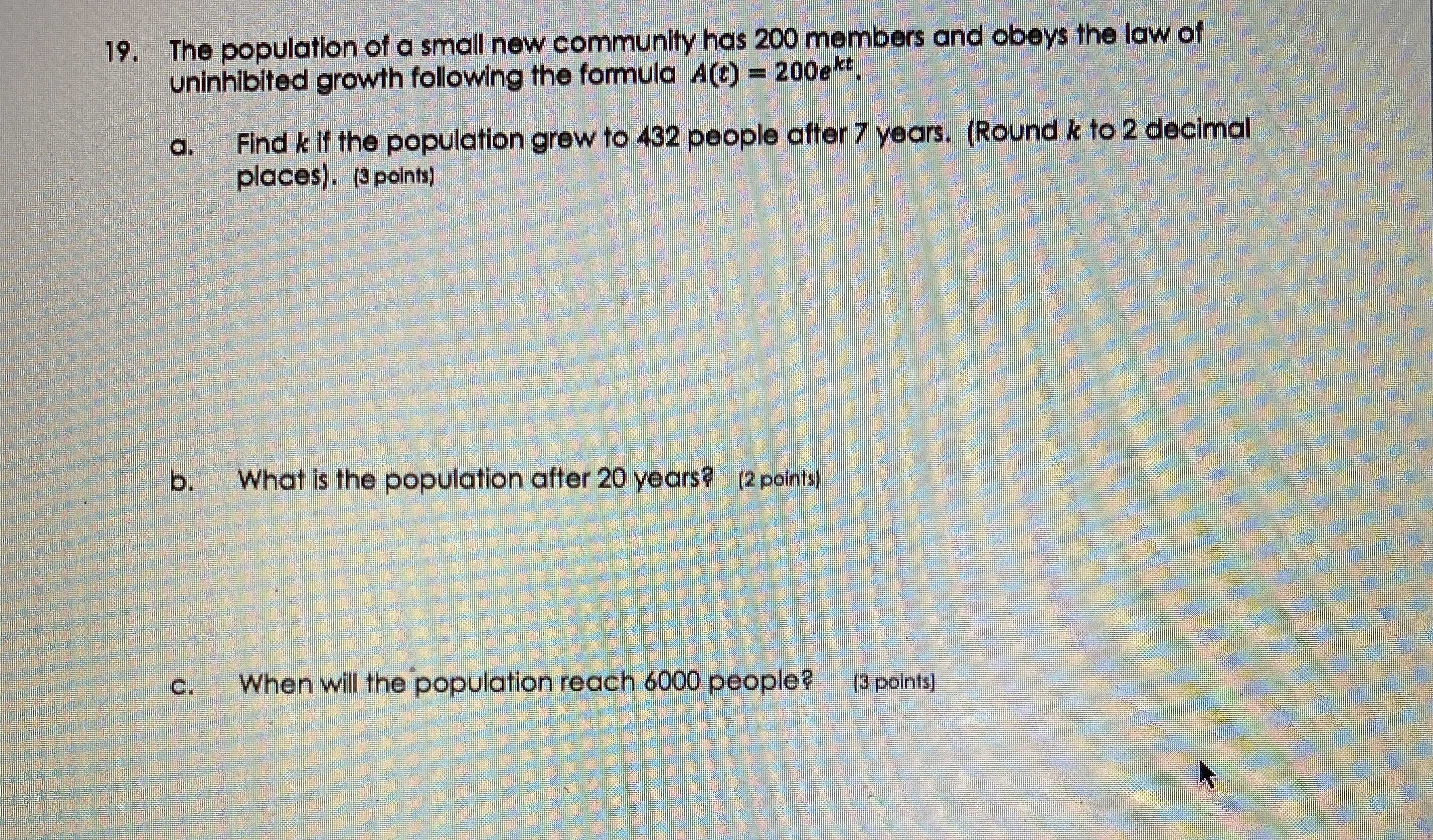### ¿Todavía tienes preguntas de matemáticas?

Pregunte a nuestros tutores expertos
Algebra
Pregunta19. The population of a small new community has $$200$$ members and obeys the law of uninhibited growth following the formula $$A ( t ) = 200 e ^ { k t }$$ ,

a. Find $$k$$ if the population grew to $$432$$ people after $$7$$ years. (Round $$k$$ to $$2$$ decimal places).(3points)

b. What is the population after $$20$$ years? (2 points)

c. When will the population reach $$6000$$ people? [3 points]

$$a.\ k= 0.11\\ b.\ A(20)= 1805\ peolple\\ c.\ t= 30.92\ years$$